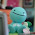## Monday, January 31, 2011

### Jocelyn Leclaire Percent Video Post

A percent can be very useful in our life today. If we didn't have a percents in our life, our lives and business would be just horrible! It would be confusing, and well just plain out dufficult. One of the ways and the most easiest way to figure out a percent is to figure out the "silver bullet" as Mr.Harbeck calls it of a number.

Cylinder & Volume Post.Height = 10cm
Diameter = 20.3cm

r= d/2
r = 20.3 / 2
r = 10.5cm

v = π x r x r x h

v = 3.14 x 10.15 x 10.15

v = 323.49cm2
v = 323.49 x 10

v = 3234.9cm3Height = 10m
Diameter = 0.8 (inside) and 1 (outside)

Inside -
R = d/2
R = 0.8/2
R = 0.4

V = π x r x r x h
V = 3.14 x 0.4 x 0.4 x h
V = 0.5 x 10
V = 5m

Outside -
R = d/2
R = 1/2
R = 0.5

V = π x r x r x h
V = 3.14 x 0.5 x 0.5 x h
V = 0.78 x 10
V = 7.8m

1.2.